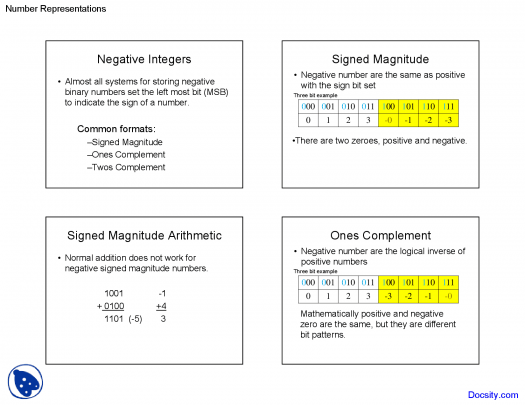11 Questions | Total Attempts: 116SettingsJust like the name indicates, almost integers are numbers that are not integer but very close to one. It is used in several branches of mathematics and you've probably used them or heard of them before. Try our quiz to verify if you have enough knowledge about these numbers.

• 1.
What's a modular form?
• A.

It is a function on the upper half plane satisfying a certain kind of functional equation

• B.

It is an analytic function on the upper half plane satisfying a certain kind of functional equation

• C.

It is a function on the lower half plane satisfying a certain kind of functional equation

• D.

It is a function on the upper plane satisfying all kinds of functional equation

• 2.
In which branch of mathematics can they be found?
• A.

Algebra

• B.

Geometry

• C.

Probabilities

• D.

Recreational mathematics

• 3.
What is an integer?
• A.

It is numbers ranked between 10 and 9

• B.

It is numbers ranked between 1 and 9

• C.

It is a number that can be written without a fractional component

• D.

It is a number inferior to 100

• 4.
What are the well-known examples of almost integers?
• A.

Logarithms

• B.

High powers of golden ratio.

• C.

Fractions

• D.

Cardinals

• 5.
What's the particularity of a golden ratio?
• A.

It is that it is multiple of 5

• B.

It is that it is lower than 100

• C.

It's that it is a Piso-Vijayaraghavan number

• D.

It is that it is higher than 100

• 6.
What is a Pisot-Vijayaraghavan number?
• A.

It is a real algebraic number greater than 10

• B.

It is a real algebraic number greater than 5

• C.

It is a real algebraic number greater than 1

• D.

It is a real algebraic number greater than 2

• 7.
What ratios are known to make almost integers?
• A.

The ratios of Fibonacci

• B.

The ratios of Mancini

• C.

The ratios of Newton

• D.

The ratios of Einstein

• 8.
From where did the ratios of Fibonacci originated from?
• A.

Italy

• B.

Indian mathematics

• C.

Chinese Algebra

• D.

Germany

• 9.
What's an Eisenstein series?
• A.

They are numerical forms with infinite series

• B.

They are modular forms with infinite series

• C.

They are particular modular forms with infinite series

• D.

They are particular modular forms with limited series

• 10.
What's a modular form?
• A.

It is a function on the upper half plane satisfying a certain kind of functional equation

• B.

It is an analytic function on the upper half plane satisfying a certain kind of functional equation

• C.

It is a function on the lower half plane satisfying a certain kind of functional equation

• D.

It is a function on the upper plane satisfying all kinds of functional equation

• 11.
What is a mathematical coincidence?
• A.

It is one that occurs when 6 expressions show a near-equation which has no theoretical explanation

• B.

It is one that occurs when 3 expressions show an equation which has no theoretical explanation

• C.

It is one that occurs when 2 expressions show a near-equation which has no theoretical explanation

• D.

It is one that occurs when 4 expressions show a near-equation which has no theoretical explanation

Related TopicsBack to top